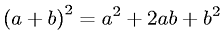Equations > Algebra > Polynomials > Square of a First Order Polynomial

### Square of a First Order PolynomialLatex Code:

MathML Code:

 ${\left(a+b\right)}^{2}={a}^{2}+2\mathrm{ab}+{b}^{2}$

MathType 5.0: